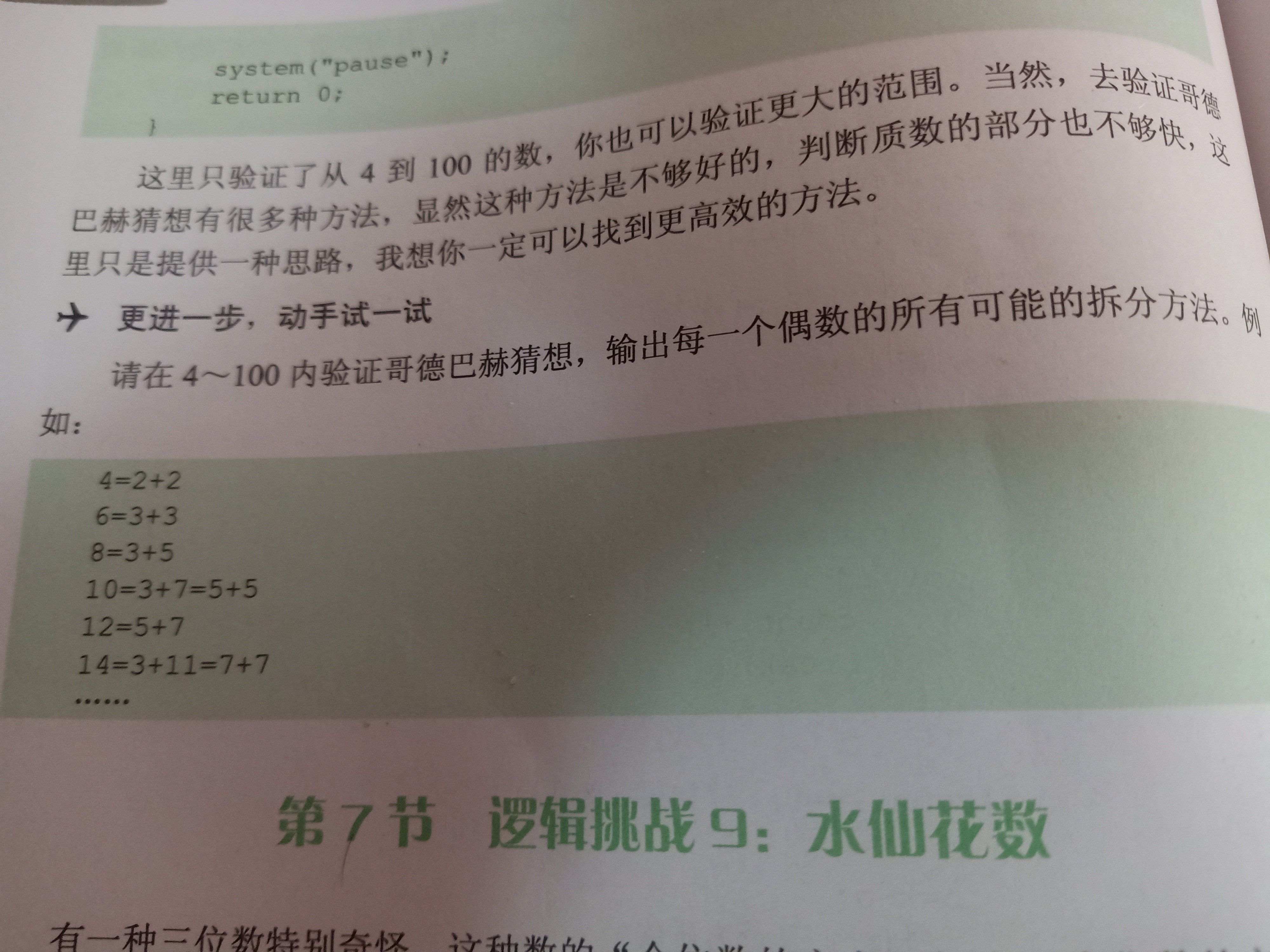2021-07-24 16:19

# 4-100内验证哥德巴赫猜想，输出每一个偶数拆分的所有可能性• 收藏

#### 1条回答默认 最新

•道标 · 落彡 2021-07-24 16:28
已采纳
``````
#include<iostream>
#include<cmath>
using namespace std;
bool sushu(int x)
{
int i = 2;
while(i <= floor(sqrt(x)) && (x % i != 0))
{
i++;
}
if(i > floor(sqrt(x)))
{
return 1;
}
return 0;
}
int main()
{
int x;
int i;
for(x = 4; x <= 100; x += 2)
{
for(i = 2; i <= x / 2; i++)
{
if(sushu(i) && sushu(x - i))
{
cout << x << "=" << i << "+" << x - i << endl;
break;
}
}
}
return 0;
}
``````
1 打赏 评论